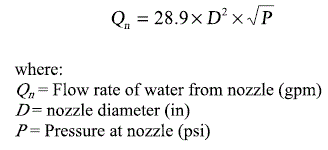You have no items in your shopping cart.
Search

# Nozzle Requirements

Find the nozzle requirements for a given pressure or flow rate.

This form is used to calculate the flow rate of a nozzle given the pressure.

Pressure:
Diameter:

Flow Rate:

## Nozzle Diameter Size

This form is used to calculate the desired diameter of a nozzle for a specific pressure and flow rate.

Pressure:
Flow Rate:

### The Equation

This calculator uses this formula to determine the Flow rate given a nozzle diameter and pressure. Or the required nozzle diameter to achieve a specified flow rate given the pressure.Reference: Washington State University